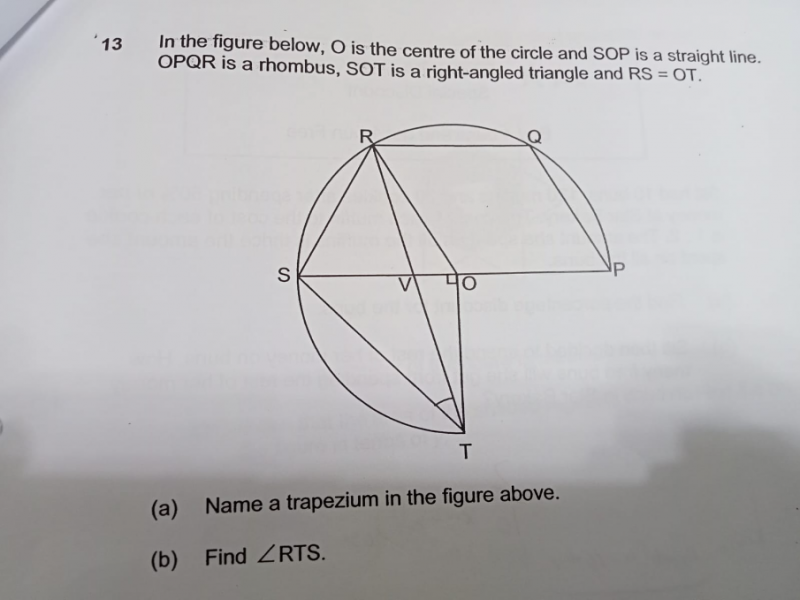# QuestionThank you

(a) PQRS is a trapezium with  SP // RQ.

(b) OQ = OR  and OP = OS and RQ is a shared line => OQRS is also a rhombus

So triangle SRO is an equilateral triangle since all 3 sides are equal, => all angles are 600

ROT = 90+60 = 150

RTO = (180-150) / 2 =15

STO = 45

Hence RTS = 45-15 = 30

0 Replies 1 Like ✔Accepted Answer